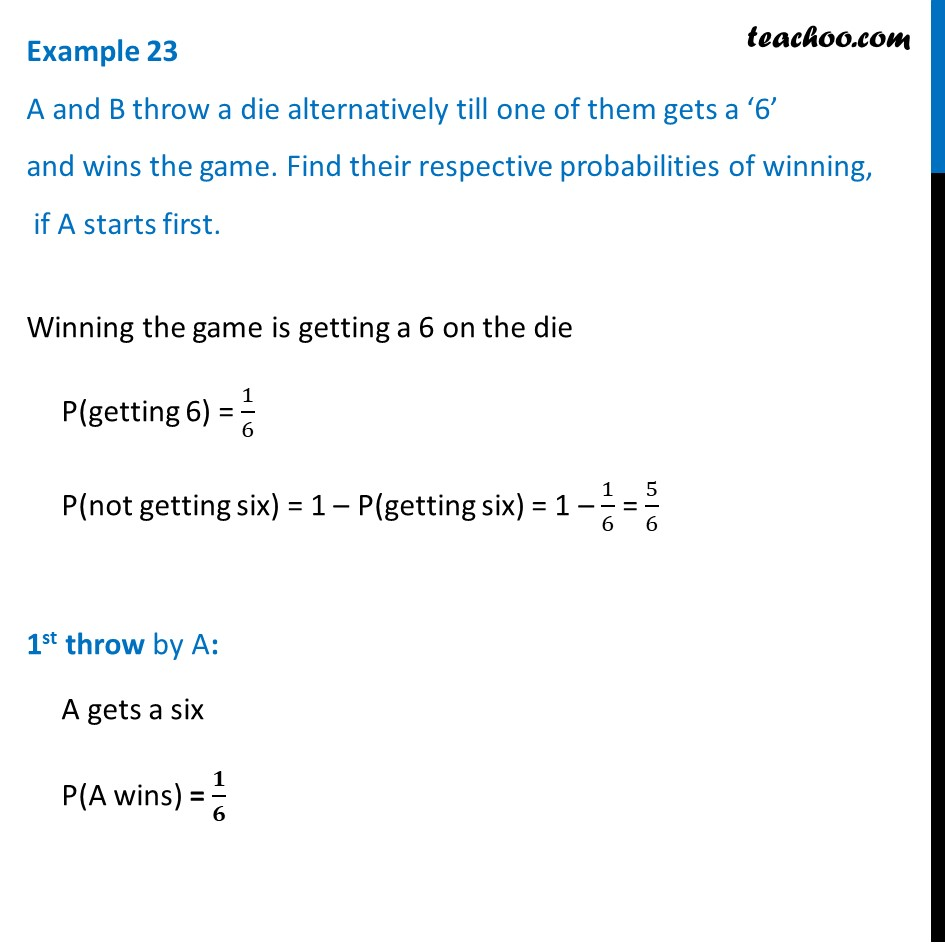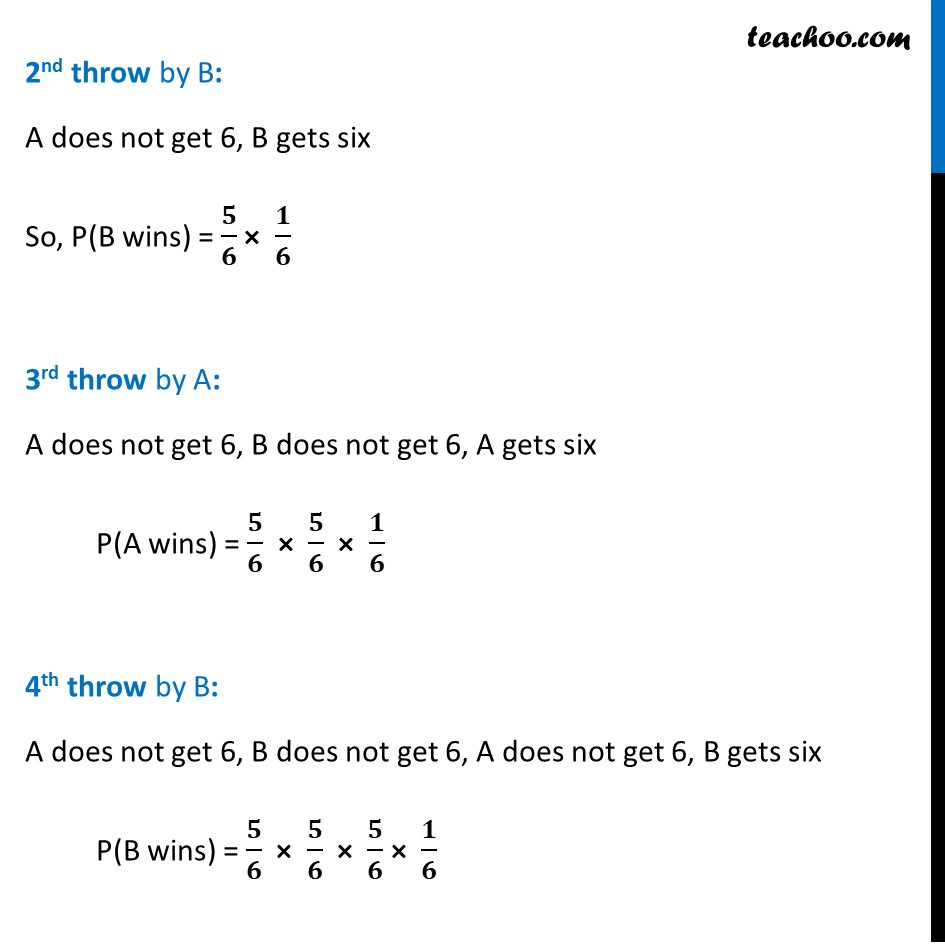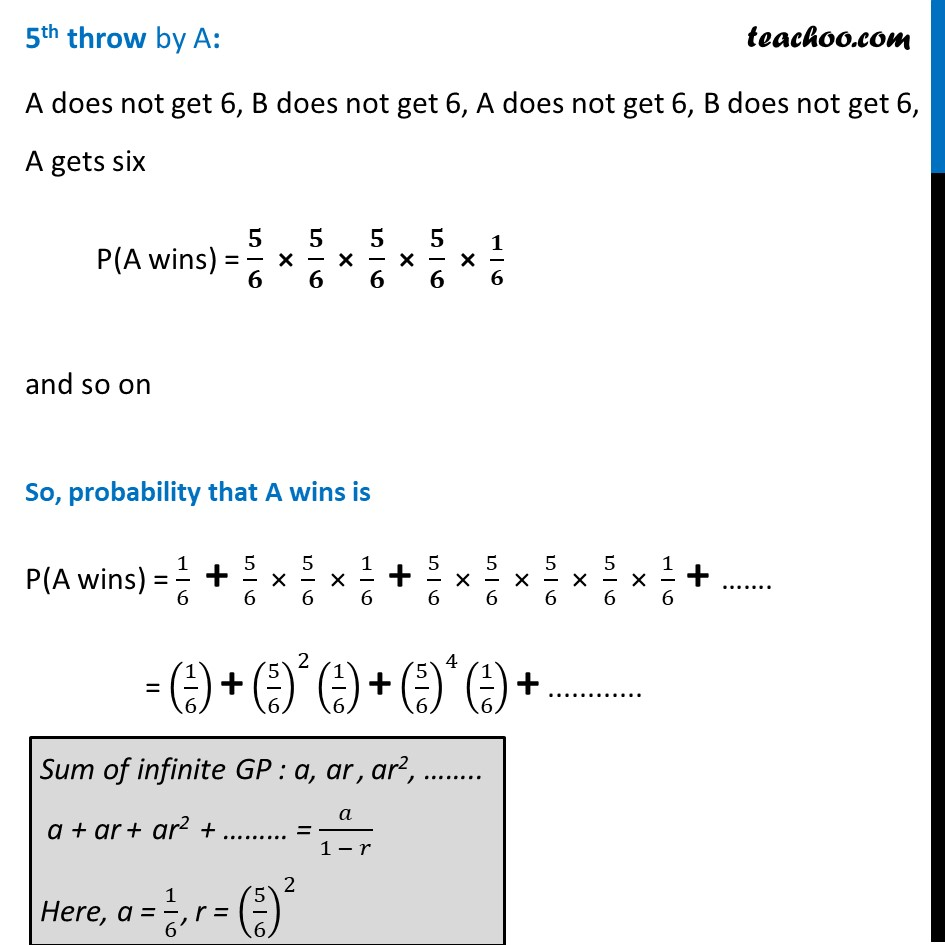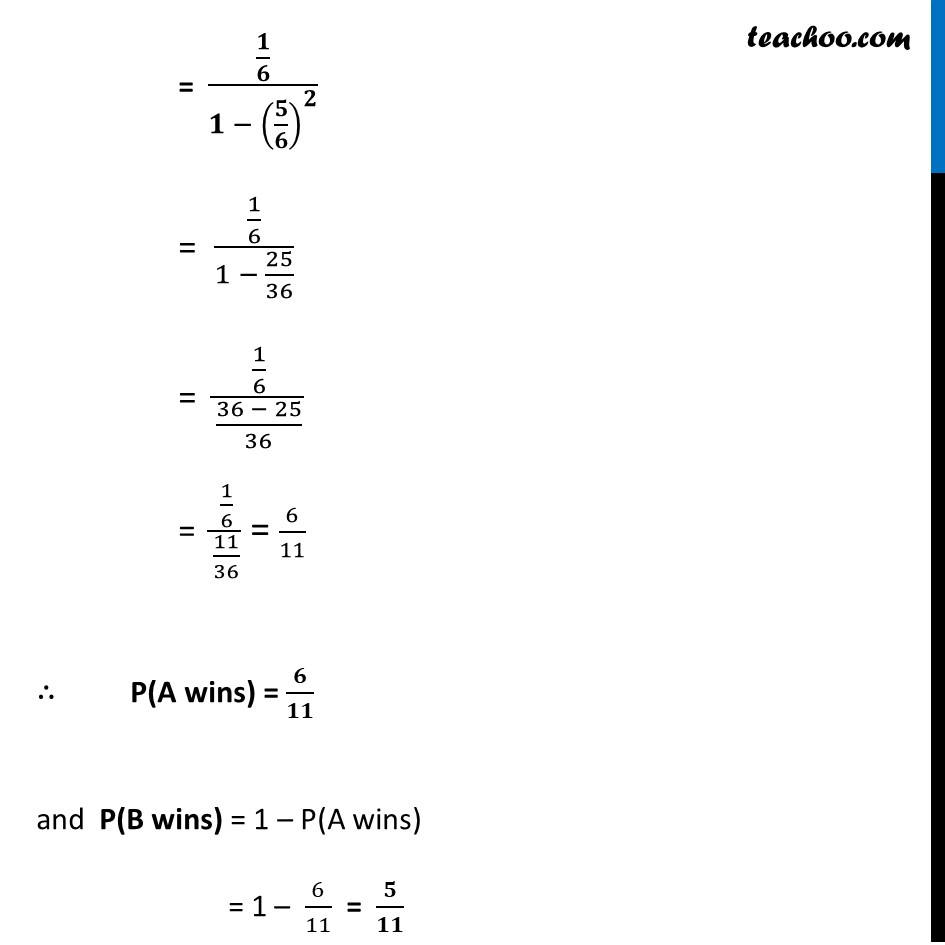Examples

Chapter 13 Class 12 Probability
Serial order wiseLearn in your speed, with individual attention - Teachoo Maths 1-on-1 Class

### Transcript

Example 23 A and B throw a die alternatively till one of them gets a ‘6’ and wins the game. Find their respective probabilities of winning, if A starts first.Winning the game is getting a 6 on the die P(getting 6) = 1/6 P(not getting six) = 1 – P(getting six) = 1 – 1/6 = 5/6 1st throw by A: A gets a six P(A wins) = 𝟏/𝟔 2nd throw by B: A does not get 6, B gets six So, P(B wins) = 𝟓/𝟔 × 𝟏/𝟔 3rd throw by A: A does not get 6, B does not get 6, A gets six P(A wins) = 𝟓/𝟔 × 𝟓/𝟔 × 𝟏/𝟔 4th throw by B: A does not get 6, B does not get 6, A does not get 6, B gets six P(B wins) = 𝟓/𝟔 × 𝟓/𝟔 × 𝟓/𝟔 × 𝟏/𝟔 5th throw by A: A does not get 6, B does not get 6, A does not get 6, B does not get 6, A gets six P(A wins) = 𝟓/𝟔 × 𝟓/𝟔 × 𝟓/𝟔 × 𝟓/𝟔 × 𝟏/𝟔 and so on So, probability that A wins is P(A wins) = 1/6 + 5/6 × 5/6 × 1/6 + 5/6 × 5/6 × 5/6 × 5/6 × 1/6 + ……. = (1/6) + (5/6)^2 (1/6) + (5/6)^4 (1/6) + ............ = (𝟏/𝟔)/(𝟏 − (𝟓/𝟔)^𝟐 ) = (1/6)/(1 − 25/36) = (1/6)/((36 − 25)/36) = (1/6)/(11/36) = 6/11 ∴ P(A wins) = 𝟔/𝟏𝟏 and P(B wins) = 1 – P(A wins) = 1 – 6/11 = 𝟓/𝟏𝟏